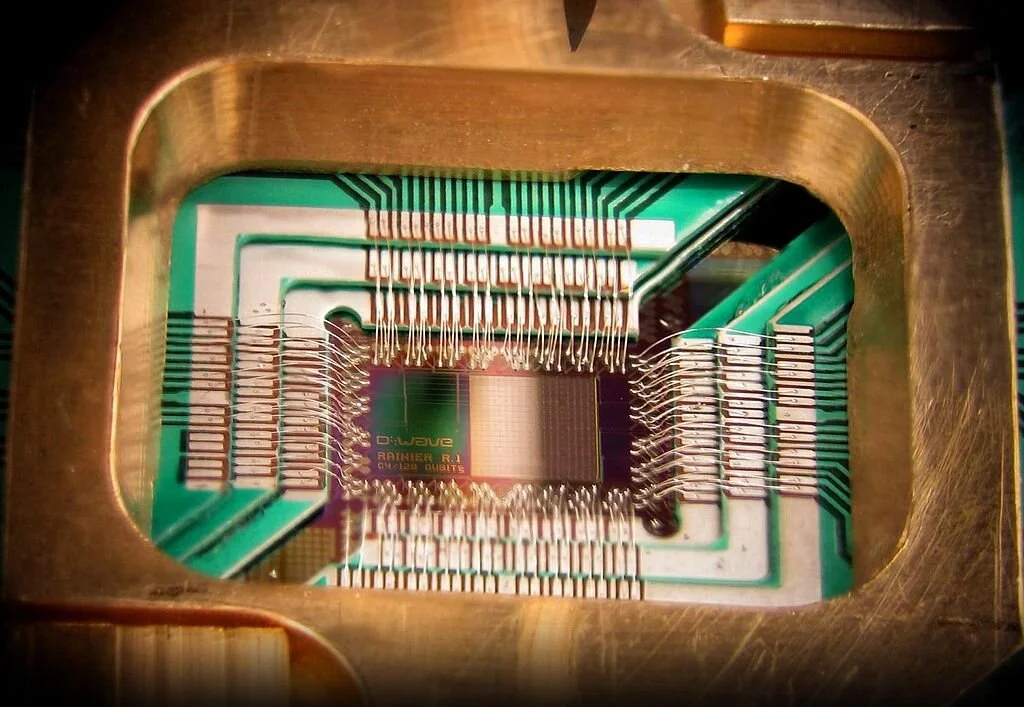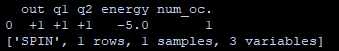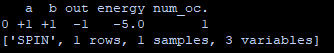# Quantum Programming 101: D-Wave’s Systems Programming Tutorial

## Introduction

D-Wave can be thought of as a pioneer in commercial Quantum Computing and is touted as one of the worlds first companies to sell Quantum Computers. In fact back in May 2011 they released the worlds first commercial quantum computer called the D-Wave One which has a 128 qubit processor.

Since then they have created hugely powerful processors by comparison. Their latest system the D-Wave 2000Q has 2000 qubits.

This tutorial will show you how to start programming for D-Waves systems by implementing logic gates on the processor as a simple example.

## HardwareD-Wave processors have some similarities and differences compared to IBM’s processors as well as Google’s. All make use of superconducting qubits that need to be cooled to very low temperatures (around -273 degrees Celsius for D-Waves Processors).

However the superconducting qubits are different in that D-Wave implements flux qubits which makes use of superconducting loops of a metal called niobium. This loop of metal allows current to flow clockwise or anticlockwise which is how information is encoded on the qubits.

IBM’s quantum computers make use of charge qubits which encode information on the charge states of the qubit.

D-Waves processors are also unique in that they were developed to solve problems using quantum annealing as opposed to solving problems with quantum logic gates like with IBM’s processors. quantum computers annealing works by first encoding your problem in to a search space of candidate solutions of which the most optimal solution is the one that requires the lowest energy.

D-Wave’s processor finds this optimal solution by going through all the possible solutions simultaneously with superposition to find the one with the lowest energy.

## Programming on D-wave Systems

To start programming on D-Wave’s Systems you will need Python and the D-Wave SDK:

## INSTALLATION

• First install Python and make sure that pip is installed

• Next open up Command Prompt and type in: pip install dwave-neal

## AND GATE IMPLEMENTATION

To solve a problem on D-Waves Systems we first have to encode the problem on to the qubits. For our example we are going to program the AND gate on to the qubits.

An AND gate is a classical logic gate consisting of 2 inputs and an output. As such we will need 3 qubits to implement this gate.

Next we need to encode a value called a H bias on the qubits. This is just the weighting value that determines whether it’s state will be a +1 or a -1 (+1 is just a binary 1 and -1 is a binary 0). To get a +1 for example the H bias need to be a negative number. To get a -1 the H bias needs to be positive. The closer the H bias is to 0 however the more likely it’s final state could be a +1 or a -1 with equal probability (a superposition).

For the AND gate the two input qubits will be q1 and q2 respectively with both having a H bias of -2. The output qubit simply called ‘out’ will have a value of 1.

``````e = -2
d = -2

h = {'q1': d, 'q2': e, 'out':1}
J = {('q1', 'out'): -1,('q2','out'):-1}``````

Next we need to encode the coupling values between the input qubits and the output qubits. The coupling values are known as the J bias.

Here we will need to set the J bias to -1 with the following code:

``J = {('q1', 'out'): -1,('q2','out'):-1}``

Where (‘q1’, ‘out’): -1 is specifying the J bias between q1 and out as -1 and (‘q2′,’out’):-1 for q2 and out.

After this we run the program and print the results with the following code:

``````response = sampler.sample_ising(h, J)
print(response)``````

After you have run the program you will get the following output. Notice how the out qubit is +1 when q1 and q2 and both +1.## NAND GATE IMPLEMENTATION

To create a NAND gate we simply leave the input H biases alone and instead invert the J bias between the inputs and outputs to 1. The H bias for out will need to be inverted to -1.

``h = {'q1': d, 'q2': e, 'out':-1}``
``J = {('q1', 'out'): 1,('q2','out'):1}``

When you run this you will get the following output:## FULL CODE

``import neal``
``sampler = neal.SimulatedAnnealingSampler()``
``#AND GATE``
``print("AND GATE")``
``e = -2``
``d = -2``
``h = {'a': d, 'b': e, 'out':1}``
``J = {('a', 'out'): -1,('b','out'):-1}``
``response = sampler.sample_ising(h, J)``
``print(response)``
``#NAND GATE``
``print("NAND GATE")``
``e = -2``
``d = -2``
``h = {'a': d, 'b': e, 'out':-1}``
``J = {('a', 'out'): 1,('b','out'):1}``
``response = sampler.sample_ising(h, J)``

`print(response)`

Want to learn about Quantum Programming? Head over to Quantum Computing UK: https://quantumcomputinguk.org/Macauley Cogginshttps://quantumcomputinguk.org/
Macauley Coggins is a Software Developer, Researcher, and Managing Director of Quantum Computing UK. He has experience in developing software for IBMs Quantum Computers and has a special interest in developing secure cryptographic systems with quantum hardware.